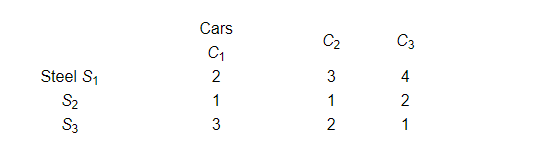# An automobile company uses three types of steel S1, S2 and S3`
Question:

An automobile company uses three types of steel $S_{1}, S_{2}$ and $S_{3}$ for producing three types of cars $C_{1}, C_{2}$ and $C_{3}$. Steel requirements (in tons) for each type of cars are given belowUsing Cramer's rule, find the number of cars of each type which can be produced using 29, 13 and 16 tons of steel of three types respectively.

Solution:

Let $x, y$ and $z$ denote the number of cars that can be produced of each type. Then,

$2 x+3 y+4 z=29$

$x+y+2 z=13$

$3 x+2 y+z=16$

Using Cramer's rule, we get

$D=\left|\begin{array}{lll}2 & 3 & 4 \\ 1 & 1 & 2 \\ 3 & 2 & 1\end{array}\right|$

$=2(1-4)-3(1-6)+4(2-3)$

$=-6+15-4$

$=5$

$D_{1}=\left|\begin{array}{lll}29 & 3 & 4 \\ 13 & 1 & 2 \\ 16 & 2 & 1\end{array}\right|$

$=29(1-4)-3(13-32)+4(26-16)$

$=-87+57+40$

$=10$

$D_{2}=\left|\begin{array}{lll}2 & 29 & 4 \\ 1 & 13 & 2 \\ 3 & 16 & 1\end{array}\right|$

$=2(13-32)-29(1-6)+4(16-39)$

$=-38+145-92$

$=15$

$D_{3}=\left|\begin{array}{lll}2 & 3 & 29 \\ 1 & 1 & 13 \\ 3 & 2 & 16\end{array}\right|$

$=2(16-26)-3(16-39)+29(2-3)$

$=-20+69-29$

$=20$

Thus,

$x=\frac{D_{1}}{D}=\frac{10}{5}=2$

$y=\frac{D_{2}}{D}=\frac{15}{5}=3$

$z=\frac{D_{3}}{D}=\frac{20}{5}=4$

Therefore, $2 C_{1}$ cars, $3 C_{2}$ cars and $4 C_{3}$ cars can be produced using the three types of steel.